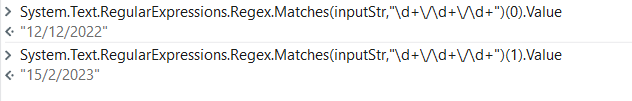# Regex to multiple dates

Hi everyone i am new to RPA and learning the regex how can i get the starting and ending dates

input = start date 12/12/2022 end date 15/2/2023

Can any one help me on this

Or you can try this way

input = start date 12/12/2022 end date 15/2/2023
1.Start Time
System.Text.RegularExpressions.Regex.matches(input,“[0-9/\-]+”)(0)

2.EndTime
System.Text.RegularExpressions.Regex.matches(input,“[0-9/\-]+”)(1)

Hi

Welcome to UiPath forum

U can use this expression in a assign activity like this to get all the matches

date_matches = System.Text.RegularExpressions.Regex.Matches(“input string”, “\d{1,2}.\d{1,2}.\d{4}”)

If u want them as array then u can use this

arr_matches = date_matches.Select(function(x) x.value).ToArray

If u want them as one string with a conman between each date value

stroutput = String.Join(“,”,date_matches.Select(function(x) x.value).ToArray)

To know more about Regex refer this post

Hope this helps

``````Input=" start date 12/12/2022 end date 15/2/2023"
Startdate=System.Text.RegularExpressions.Regex.Match(Input,"(?<=start date\s)\d+\/\d+\/\d+"). Value
Enddate=System.Text.RegularExpressions.Regex.Match(Input,"(?<=end date\s)\d+\/\d+\/\d+"). Value
``````

Hope it helps!!

Hi,

There are several ways to achieve it.

Use Matches method

``````mc = System.Text.RegularExpressions.Regex.Matches(yourString,"\b\d{1,2}/\d{1,2}/\d{4}\b")
``````

Then

``````mc(0).Value

mc(1).Value
``````

Use Groups

``````m = System.Text.RegularExpressions.Regex.Match(yourString," start date (?<START>\d{1,2}/\d{1,2}/\d{4}) end date (?<END>\d{1,2}/\d{1,2}/\d{4})")
``````

Then

``````m.Groups("START").Value

m.Groups("END").Value
``````

Sample
Sample20230926-2L.zip (2.5 KB)

Regards,